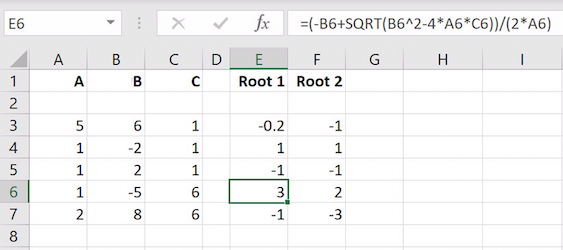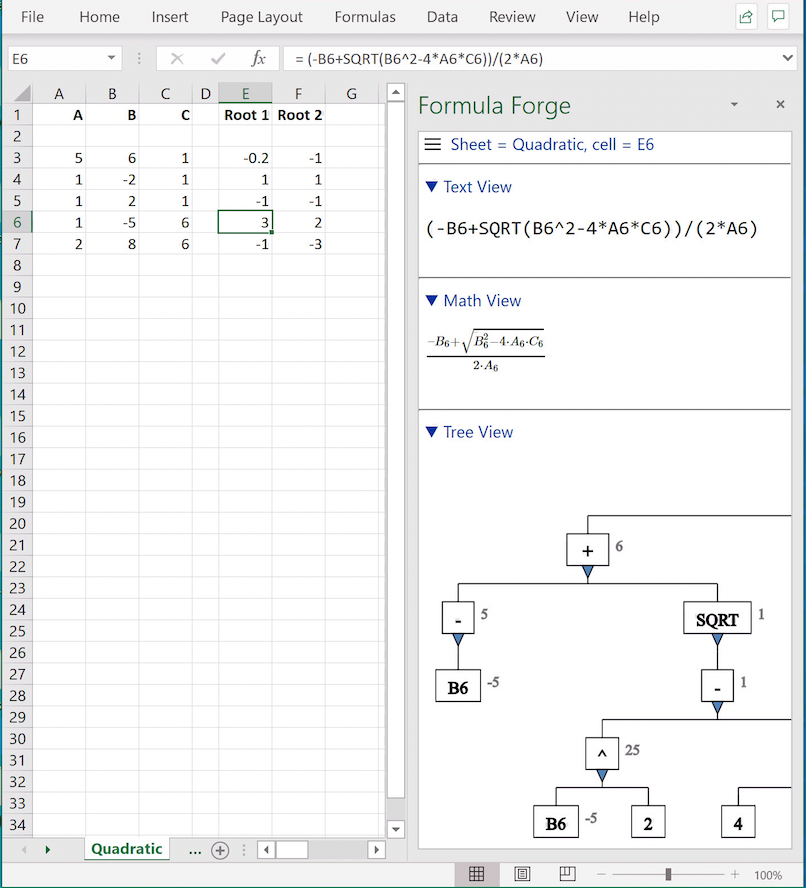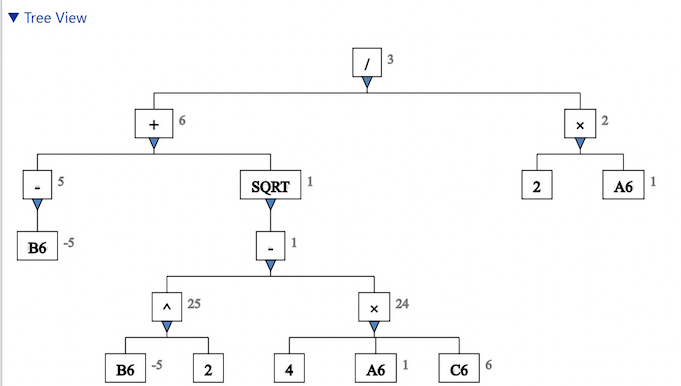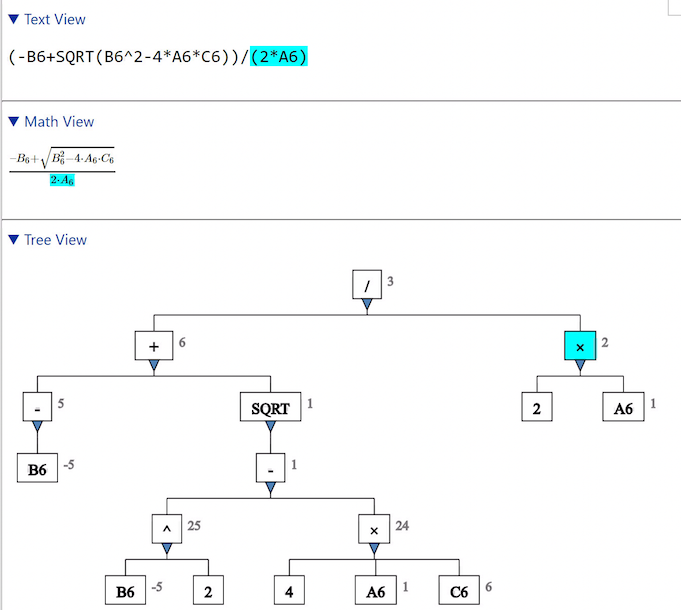# Use Cases

## Use Case: Recognizing a Formula

Formulas in spreadsheets can be hard to understand. Do you recognize the one in cell `E6`?A formula in cell E6

Let's ask Formula Forge for help. Select cell `E6` and click the `Get Formula` button on Excel's `Formulas` ribbon to see a pane with three views of the formula in cell `E6`. The math view, which shows the formula as it might be typeset in a book, is particularly informative: it reveals that this formula is none other than the familiar quadratic formula taught in high school algebra.The `Formula Forge` pane

Along with the math view are a text view and a tree view. The `Formula Forge ` pane is not wide enough to display the entire tree view, so we click its left edge and drag it left to widen the pane.The widened tree view

Now we can see the entire tree view. This view displays the elements that comprise the formula, according to the order in which they are evaluated, from the bottom up. The elements at the bottom are constants or references, and the elements higher up correspond to operators or functions.

To understand where an element in the tree view occurs in the entire formula, we can click that element to highlight it in all views. To find the smallest element in the tree view that contains a symbol in either the text view or the math view, we can click that symbol to highlight the containing element in all views. Formula Forge automatically synchronizes all three views when an action occurs in any one of those views.Selecting an element